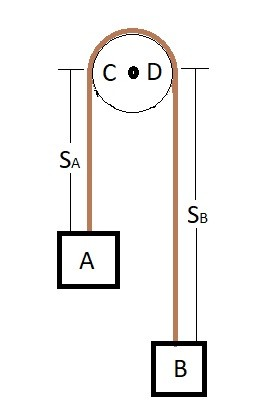# Dependent Motion

In certain cases the motion of one particle could be dependent on the motion of another particle.  For example if you had two objects connected to each other through a cord their motion would be dependent on each other.  You could further this example by wrapping the cord around a pulley or a series of pulleys as seen in the image below.In the image above the lengths $s_A$ and $s_B$ are dependent on the positions of particle A and particle B.  If you were to pull particle B down particle A will move upward.  While on the other hand if you were to move particle A down particle B will move upward.   This is dependent motion.

To determine how far each particle will move you will need to determine the length of the chord.  You will need to find the length $S_A$ as well as the length $S_B$.  Remember these two lengths will be dependent on each.  To find the relationship of these two lengths use the equation below.

Eq 1 $s_A + l_{CD} + s_B = l_t$

Previous

|